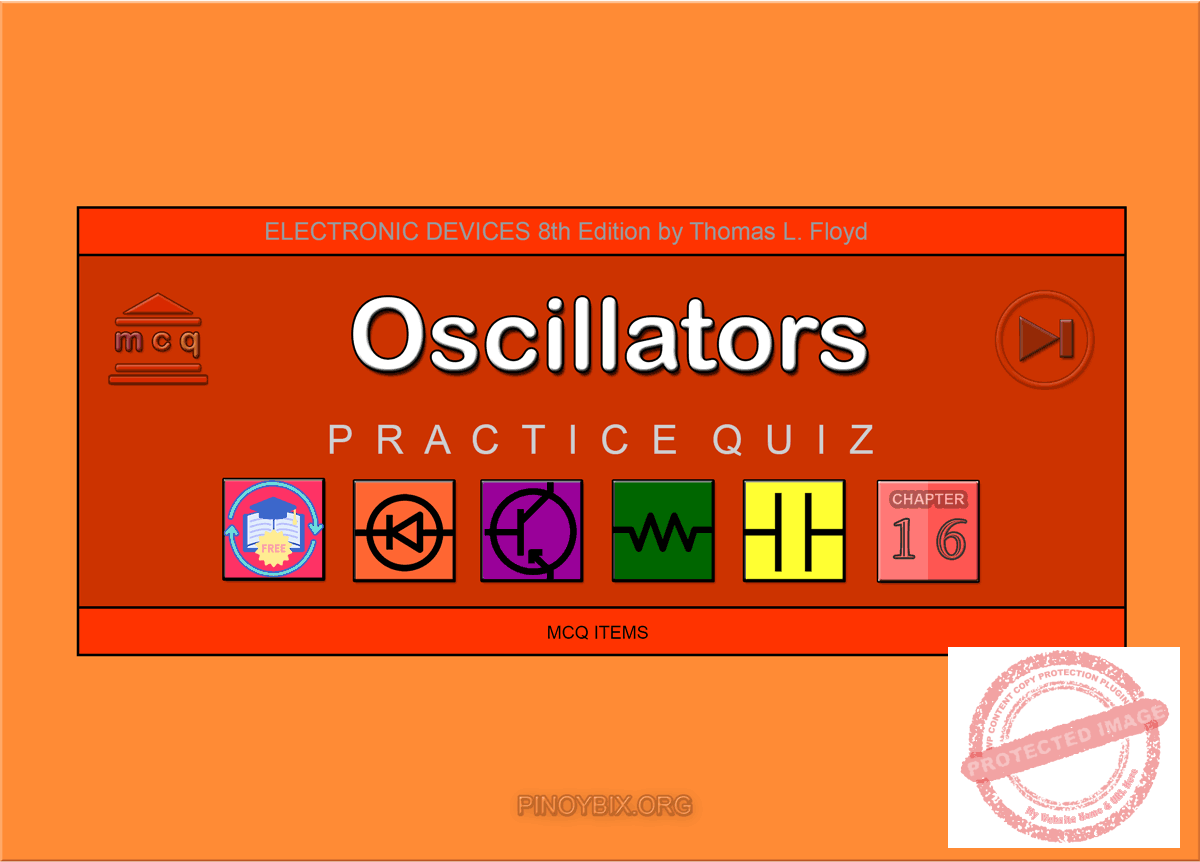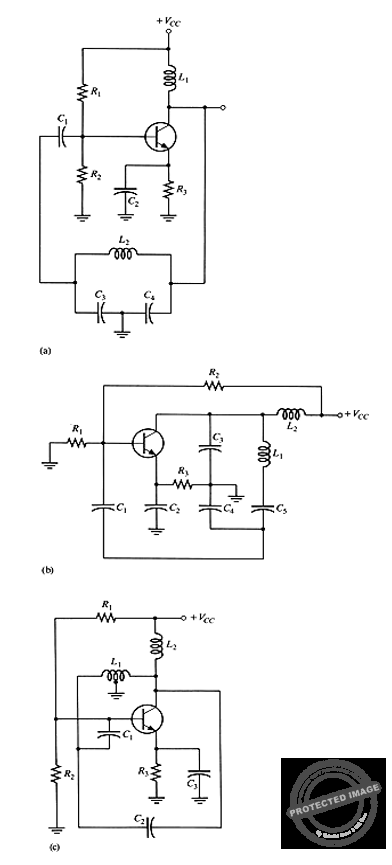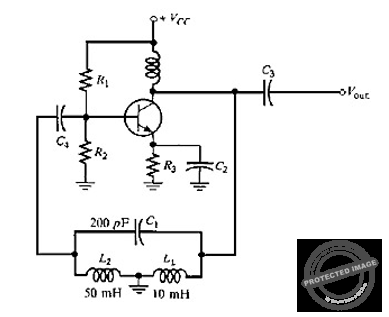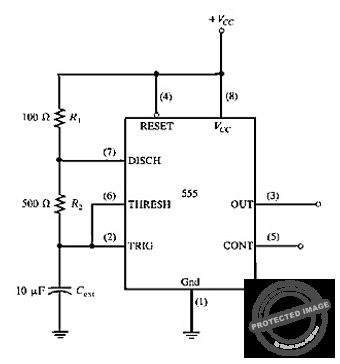# Floyd: MCQ in Oscillators

(Last Updated On: December 9, 2019)This is the Multiple Choice Questions in Oscillators from the book Electronic Devices – Electron Flow Version and Conventional Current Version 8th Edition by Thomas L. Floyd. If you are looking for a reviewer in Electronics Engineering this will definitely help. I can assure you that this will be a great help in reviewing the book in preparation for your Board Exam. Make sure to familiarize each and every questions to increase the chance of passing the ECE Board Exam.

If you are looking for the Self-test in Floyd’s Electronic Devices proceed to

#### Practice Exam Test Questions

Choose the letter of the best answer in each questions.

1. In order to start up, a feedback oscillator requires

A. negative feedback less than 1.

B. positive feedback greater than 1.

C. unity feedback equal to 1.

D. no feedback.

Solution:

2. What is the total phase shift requirement, around the feedback loop, for a phase-shift oscillator?

A. 90°

B. 180°

C. 270°

D. 360°

Solution:

3. What is the relationship between the series and parallel resonant frequencies of a quartz crystal?

A. They are equal.

B. Parallel resonant frequency is approximately 1 kHz higher than series resonant frequency.

C. Series resonant frequency is approximately 1 kHz higher than parallel resonant frequency.

D. none of the above

Solution:

4. At series resonance, the impedance of a crystal is

A. minimum.

B. maximum.

C. equal.

D. zero.

Solution:

5. At parallel resonance, the impedance of a crystal is

A. minimum.

B. maximum.

C. equal.

D. zero.

Solution:

6. What is the minimum frequency at which a crystal will oscillate?

A. seventh harmonic

B. third harmonic

C. fundamental

D. second harmonic

Solution:

7. Refer to Figure 16-1(a). This circuit is known asFigure 16-1

A. a Clapp oscillator.

B. an Armstrong oscillator.

C. a Colpitts oscillator.

D. a Hartley oscillator.

Solution:

8. Refer to Figure 16-1(b). This circuit is known as

A. a Clapp oscillator.

B. an Armstrong oscillator.

C. a Colpitts oscillator.

D. a Hartley oscillator.

Solution:

9. Refer to Figure 16-1(c). This circuit is known as

A. a Clapp oscillator.

B. an Armstrong oscillator.

C. a Colpitts oscillator.

D. a Hartley oscillator.

Solution:

10. Refer to Figure 16-1(b). The resonant frequency is controlled by

A. C3 and L1.

B. C2, C4, C5, and L1.

C. C3, C4, C5, and L1.

D. C3, C4, C5, and L2.

Solution:

11. Calculate the fr of a lead-lag network if R1 = R2 = 6.8 kΩ, and C1 = C2 = 0.05 µF.

A. 468 Hz

B. 4.68 kHz

C. 46.8 kHz

D. 468 kHz

Solution:

12. Refer to Figure 16-2. Calculate the resonant frequency.Figure 16-2

A. 1.126 kHz

B. 6.17 kHz

C. 23.9 MHz

D. 14.1 MHz

Solution:

13. Refer to Figure 16-3. Determine the resonant frequency.Figure 16-3

A. 123.4 kHz

B. 61.7 kHz

C. 45.94 kHz

D. 23.1 kHz

Solution:

14. Refer to Figure 16-3. If C1 increases in value, the resonant frequency will

A. increase.

B. decrease.

C. remain the same.

D. none of the above

Solution:

15. A certain oscillator has a tap on the inductor in the tank circuit. This oscillator is probably

A. a Colpitts oscillator.

B. a Clapp oscillator.

C. a crystal oscillator.

D. a Hartley oscillator.

Solution:

16. An op-amp integrator has a square-wave input. The output should be

A. a sine wave.

B. a triangle wave.

C. a square wave.

D. pure dc.

Solution:

17. Refer to Figure 16-4. This circuit isFigure 16-4

A. a sine-wave oscillator.

B. a monostable multivibrator.

C. an astable multivibrator.

D. a VCO.

Solution:

18. Refer to Figure 16-4. Determine the frequency of oscillation, if any.

A. 131 Hz

B. 262 Hz

C. 2.62 kHz

D. none

Solution:

19. Refer to Figure 16-4. What is the duty cycle, if any?

A. 16.3%

B. 54.5%

C. 86.9%

D. none

Solution:

20. Refer to Figure 16-4. Assuming it is configured as an oscillator and if you desired to reduce the duty to less than 50%, the following circuit change would need to be made.

A. Reduce the size of R1.

B. Reduce the size of R2.

C. Increase the size of R1.

D. Connect a diode in parallel with R1.

Solution:

21. A circuit that can change the frequency of oscillation with an application of a dc voltage is sometimes called

A. a voltage-controlled oscillator.

B. a crystal oscillator.

C. a Hartley oscillator.

D. an astable multivibrator.

Solution:

22. Sinusoidal oscillators operate with _____ feedback.

A. positive

B. negative

c. either of the two

D. neither of the two

Solution:

23. One condition for positive feedback is that the phase shift around the feedback loop must be _____°.

A. 0

B. 90

C. 180

D. 45

Solution:

24. The start-up gain of an oscillator must be _____ one.

A. equal to

B. less than

C. greater than

D. none of the above

Solution:

25. For frequencies up to 1 MHz, the _____ is the most widely used type of sinusoidal RC oscillator.

A. Wien-bridge

B. phase-shift

C. twin-T

D. none of the above

Solution:

26. The lead-lag circuit in the Wien-bridge oscillator has a resonant frequency at which the attenuation is

A. 1/2.

B. 1/4.

C. 1/3.

D. 1/5.

Solution:

27. The attenuation of the three-section RC feedback phase-shift oscillator is

A. 1/9.

B. 1/30.

C. 1/3.

D. 1/29.

Solution:

28. The twin-T oscillator produces a _____ response.

A. low-pass

B. high-pass

C. band-pass

D. band-stop

Solution:

29. The feedback signal in a(n) _____ oscillator is derived from a capacitive voltage divider in the LC circuit.

A. Hartley

B. Armstrong

C. Colpitts

D. none of the above

Solution:

30. The feedback signal in a(n) _____ oscillator is derived from an inductive voltage divider in the LC circuit.

A. Hartley

B. Armstrong

C. Colpitts

D. none of the above

Solution:

#### TRUE/FALSE

1. Oscillators operate on negative feedback.

A. True

B. False

Solution:

2. The start-up gain of an oscillator must be greater than 1.

A. True

B. False

Solution:

3. The feedback signal in a Hartley oscillator is derived from an inductive voltage in the tank circuit.

A. True

B. False

Solution:

4. Crystal oscillators are inherently unstable.

A. True

B. False

Solution:

5. The frequency in a VCO can be varied with a DC voltage.

A. True

B. False

Solution:

6. LC feedback elements are normally used in oscillators for frequencies greater than 1 MHz.

A. True

B. False

Solution:

7. The positive feedback voltage in an oscillator has no net phase shift.

A. True

B. False

Solution:

8. For a Wien-bridge oscillator, resonance occurs when Vout/Vin = 1.

A. True

B. False

Solution:

9. The feedback network in a Colpitts oscillator contains two series capacitors and a parallel inductor.

A. True

B. False

Solution:

10. In the astable mode, a 555 timer is connected as a free-running relaxation oscillator.

A. True

B. False

Solution:

### Complete List of Chapter MCQs in Floyd’s Electronic Devices

Help Me Makes a Difference!

 P inoyBIX educates thousands of reviewers/students a day in preparation for their board examinations. Also provides professionals with materials for their lectures and practice exams. Help me go forward with the same spirit. “Will you make a small \$5 gift today?” Option 1 : \$1 USD Option 2 : \$3 USD Option 3 : \$5 USD Option 4 : \$10 USD Option 5 : \$25 USD Option 6 : \$50 USD Option 7 : \$100 USD Option 8 : Other Amount© 2014 PinoyBIX Engineering. © 2019 All Rights Reserved | How to Donate? |#### GEAS Solution

Dynamics problem Economics problem Physics problem Statics problem Strength problem Thermodynamics problem

#### Questions and Answers in GEAS

Engineering Economics Engineering Laws and Ethics Engineering Management Engineering Materials Engineering Mechanics General Chemistry Physics Strength of Materials Thermodynamics
Consider Simple Act of Caring!: LIKE MY FB PAGE

Our app is now available on Google Play, Pinoybix Elex